# Roman numerals

Write numbers written in Roman numerals as decimal.

DXVI =  516
MCLXXXIII =  1183
MDCCXXXIII =  1733
MDCCCXLI =  1841
DCCCLXXI =  871
MCCCLXXXIII =  1383
MCCL =  1250
MDLXVIII =  1568
MMCXIII =  2113
MMCCLXXIV =  2274

### Step-by-step explanation:

DXVI = 516
MCLXXXIII = 1183
MDCCXXXIII = 1733
MDCCCXLI = 1841
DCCCLXXI = 871
MCCCLXXXIII = 1383
MCCL = 1250
MDLXVIII = 1568
MMCXIII = 2113
MMCCLXXIV = 2274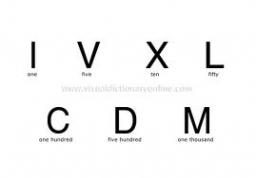Did you find an error or inaccuracy? Feel free to write us. Thank you!## Related math problems and questions:

• Roman numerals +Add up the number written in Roman numerals. Write the results as a decimal number.
• Roman numerals 2+Add up the number written in Roman numerals. Write the results as roman numbers.
• Roman numerals 2-Subtract up the number written in Roman numerals. Write the results as Roman numbers.Added together and write as decimal number: LXVII + MLXIV
• ClassmatesRoman is ranked 12th highest and eleventh lowest pupil. How many classmates does Roman have?
• Written numberPlace+values x ten thousands =30 thousands
• Mistake in expression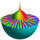While attempting to multiply the expression (2 - 5i)(5 + 2i) a student made a mistake. (2 - 5i)(5 + 2i) = 10 + 4i - 25i - 10i2 = 10 + 4(-1) - 25(-1) - 10(1) = 10 - 4 + 25 - 10 = 21 Complete the explanation and correct the error. Hint: The student inc
• DigitsWrite the smallest and largest 2-digit natural number.
• April (month)April has 30 days. Roman saves his money in the box for the whole month. Every third day you saves 10 cents. How many cents did Roman saved during the whole month when start saving on April 1?
• Star equationWrite digits instead of stars so that the sum of the written digits is odd and is true equality: 42 · ∗8 = 2 ∗∗∗
• Minutes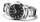Write as fraction in basic form which part of the week is 980 minutes.
• DozenWhat is the product of 26 and 5? Write the answer in an Arabic numeral. Add up the digits. How many of this is in a dozen? Divide #114 by this
• Children in the classroom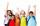How many children are in the classroom, where Ladislav is 10th lightest and 16th heaviest in class?
• Write decimalsWrite in the decimal system the short and advanced form of these numbers: a) four thousand seventy-nine b) five hundred and one thousand six hundred and ten c) nine million twenty-six
• Two houses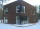There are twice as many people living in the first house as in the second house. Altogether, there are 81 of them living in both. How many people live in each house?
• SummandOne of the summands is 145. The second is 10 more. Determine the sum of the summands.
• Ten fractionsWrite ten fractions between 1/3 and 2/3